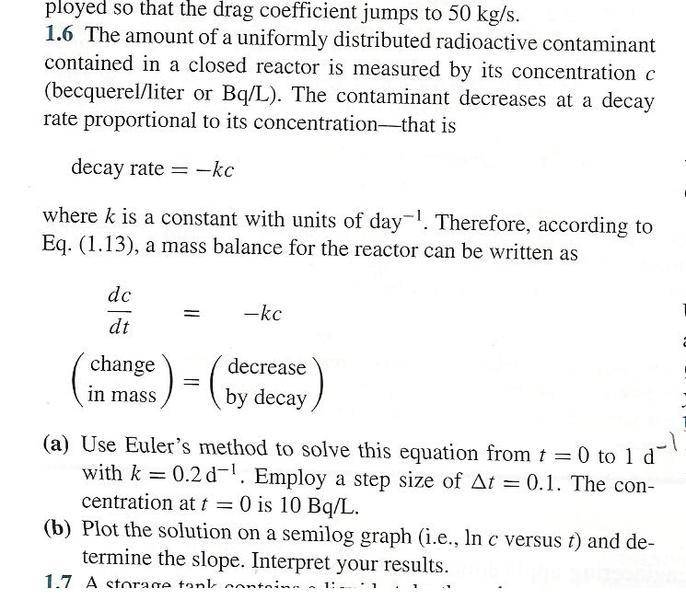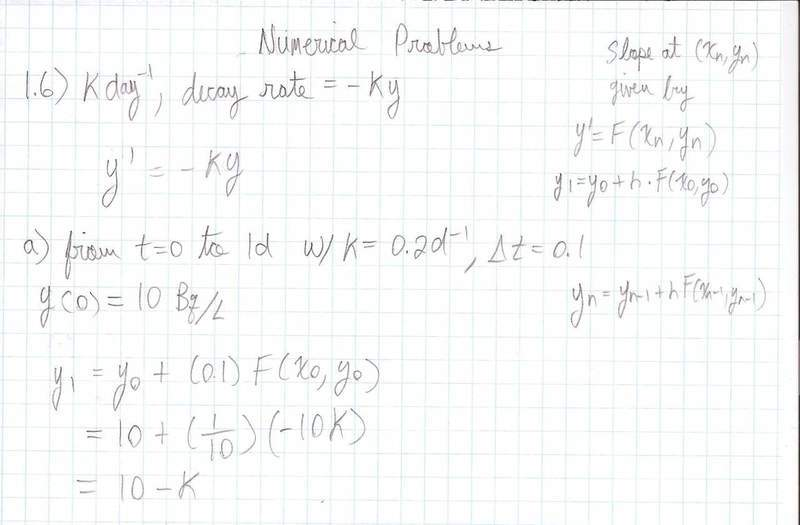# Euler's Method

jegues

## Homework Statement

See figure attached for problem statement.## The Attempt at a Solution

Here's as far as I got,The part that confuses me is the range we should solve this equation. It says,

$$\text{from } t=0 \text{ to } 1d$$

I put the $$^{-1}$$ in there with pencil because I thought it was a typo. Is it?

If I can figure out where to stop I really just have to keep repeating the formula,

$$y_{n} = y_{n-1} + \Delta t F(x_{n-1}, y_{n-1})$$

Right?

Thanks again!

## Answers and Replies

jegues
Bump, still looking for some help.

Staff Emeritus
Homework Helper
$$\text{from } t=0 \text{ to } 1d$$

I put the $$^{-1}$$ in there with pencil because I thought it was a typo. Is it?
No, t is time and it's units are days or d, not d-1.

If I can figure out where to stop I really just have to keep repeating the formula,

$$y_{n} = y_{n-1} + \Delta t F(x_{n-1}, y_{n-1})$$

Right?

Thanks again!
Yes, that is the correct approach. You stop once you have reached t = 1 day.

jegues
No, t is time and it's units are days or d, not d-1.

Yes, that is the correct approach. You stop once you have reached t = 1 day.

But how will I reach 1 day?

I have,

$$10-k$$ where k has units $$day^{-1}$$.

Can you explain please?

Staff Emeritus
Homework Helper
Well, if you're going to be careful about the units -- and it's a good thing if you are -- then you need to include the units correctly on all quantities. Note that Δt should really be 0.1d, not simply 0.1 as stated in the problem statement.

So you really have

y1 = y0 - k·y0·Δt
. . .= 10 Bq/L - (0.2 d-1)*(10 Bq/L)*(0.1 d)
. . .= 10 Bq/L - 0.2 Bq/L = 9.8 Bq/L

That is y1, so that is the concentration at 1·Δt or 0.1 days.
When you calculate yn, you will have the concentration after n·Δt or n·0.1 days.